# ISEE Upper Level Math : How to find the area of a parallelogram

## Example Questions

### Example Question #1 : How To Find The Area Of A Parallelogram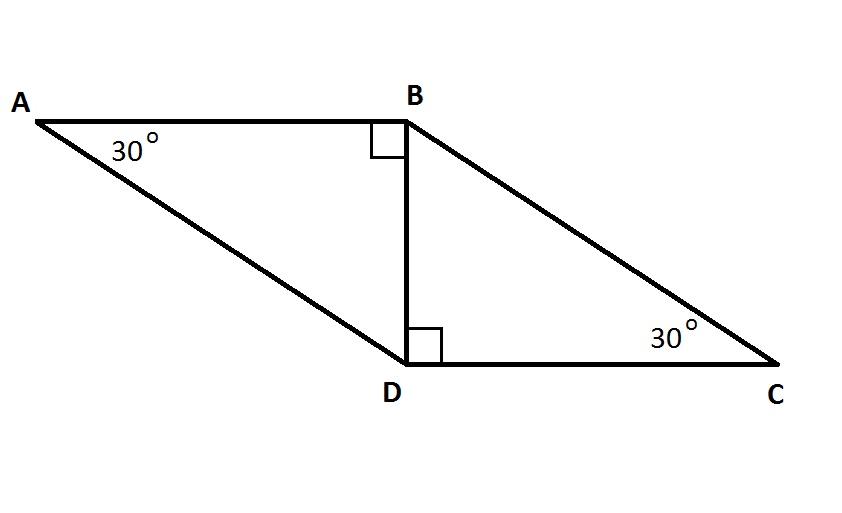Give the area of the above parallelogram if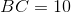.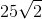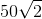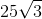Explanation:

Multiply height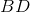by base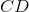to get the area.

By the 30-60-90 Theorem: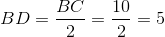and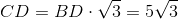The area is therefore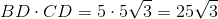### Example Question #2 : How To Find The Area Of A Parallelogram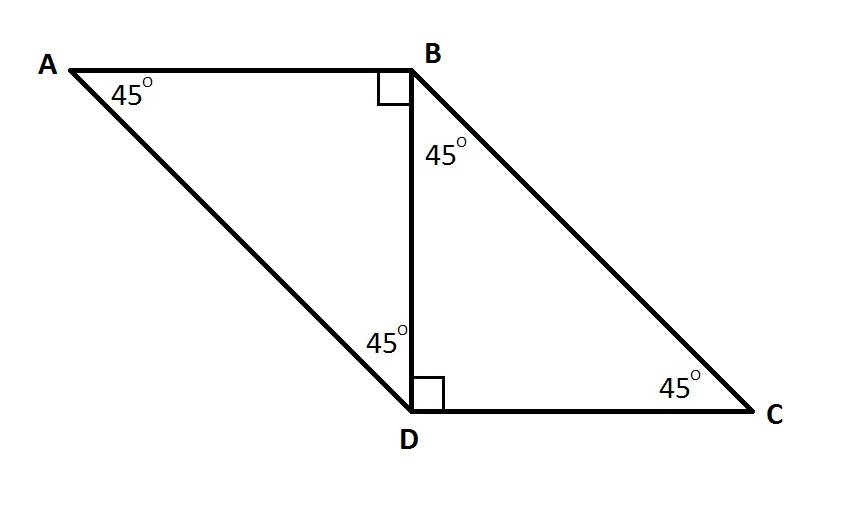Give the area of the above parallelogram if.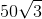Explanation:

Multiply heightby baseto get the area.

By the 45-45-90 Theorem,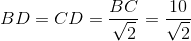The area is therefore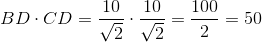### Example Question #3 : How To Find The Area Of A Parallelogram

Three of the vertices of a parallelogram on the coordinate plane are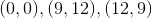. What is the area of the parallelogram?Insufficient information is given to answer the problem.Explanation:

As can be seen in the diagram, there are three possible locations of the fourth point of the parallelogram: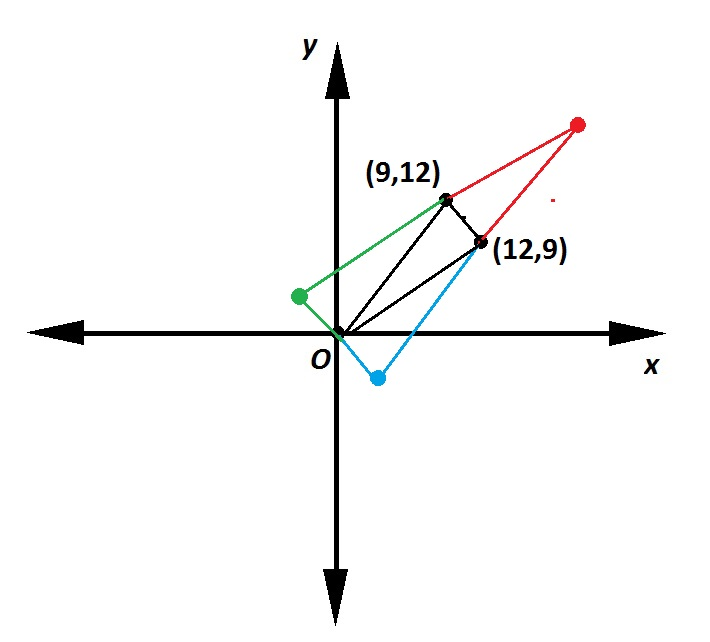Regardless of the location of the fourth point, however, the triangle with the given three vertices comprises exactly half the parallelogram. Therefore, the parallelogram has double that of the triangle.

The area of the triangle can be computed by noting that the triangle is actually a part of a 12-by-12 square with three additional right triangles cut out: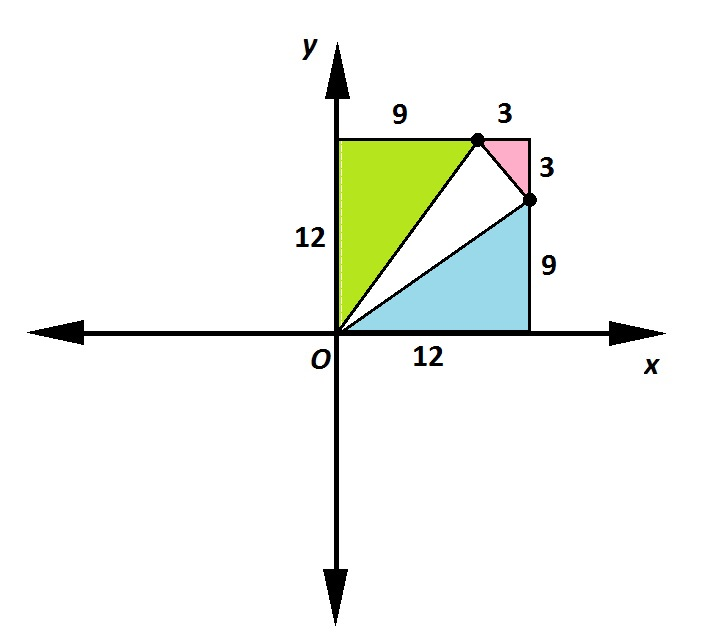The area of the 12 by 12 square is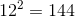The area of the green triangle is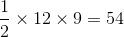.

The area of the blue triangle is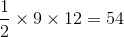.

The area of the pink triangle is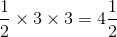.

The area of the main triangle is therefore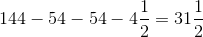The parallelogram has area twice this, or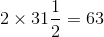.

### Example Question #4 : How To Find The Area Of A Parallelogram

One of the sides of a square on the coordinate plane has an endpoint at the point with coordinates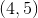; it has the origin as its other endpoint. What is the area of this square?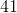Explanation:

The length of a segment with endpoints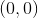andcan be found using the distance formula with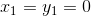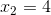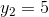: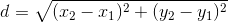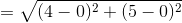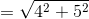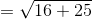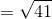This is the length of one side of the square, so the area is the square of this, or 41.